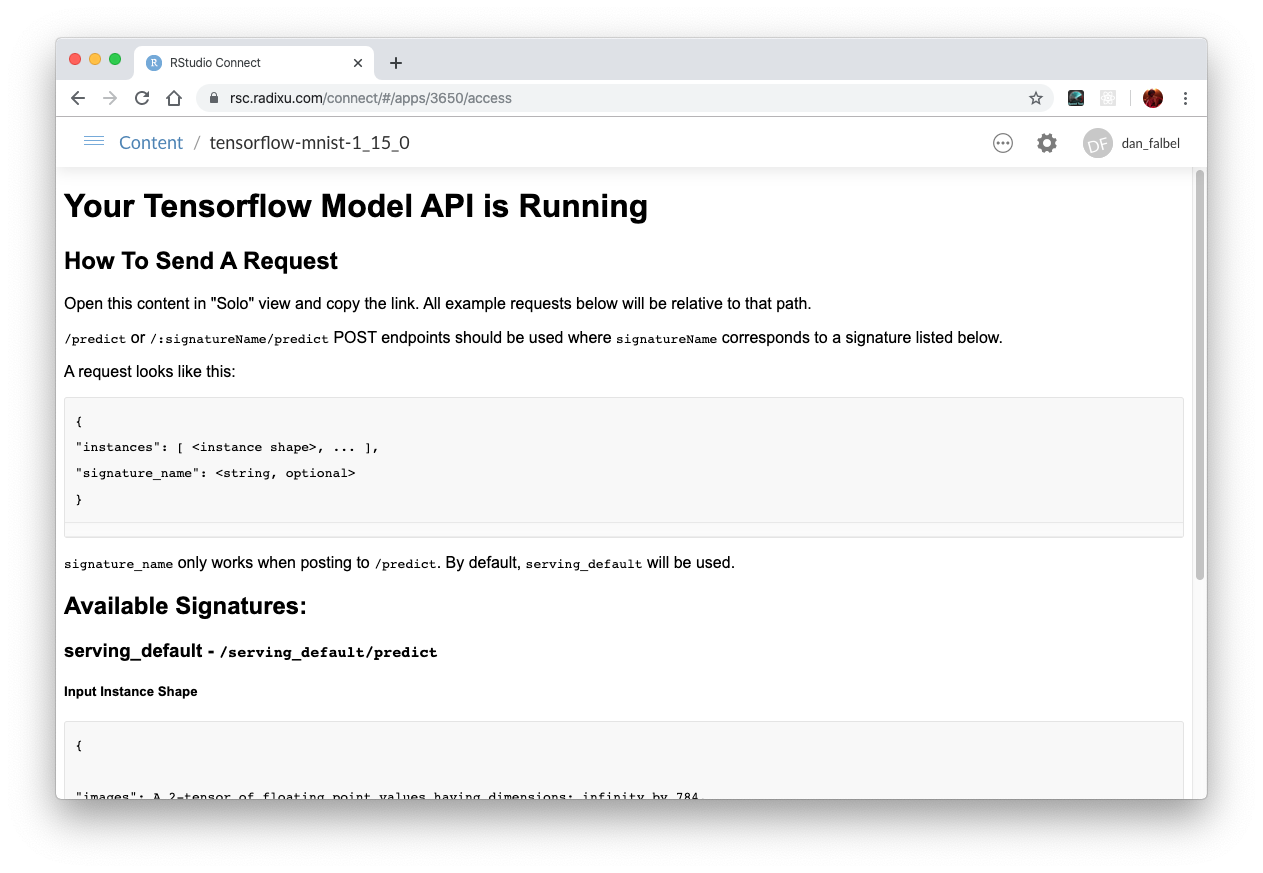# Deploying a TensorFlow Model to RStudio Connect

## Deprecation Notice

Starting in RStudio Connect 2022.09.0, TensorFlow model hosting is deprecated and will be removed in an upcoming release. Use an API framework like Plumber, Flask, or FastAPI to create an HTTP API for your TensorFlow model.

In this tutorial you will learn how to deploy a TensorFlow model to RStudio Connect. RStudio Connect uses TensorFlow Serving for performance but makes it much easier for R users to manage their deployment.

## Building the model

The first thing we are going to do is to build our model. We will use the Keras API to build this model.

We will use the MNIST dataset to build our model.

``````library(keras)
library(tensorflow)
mnist <- dataset_mnist()

mnist\$train\$x <- (mnist\$train\$x/255) %>%
array_reshape(., dim = c(dim(.), 1))

mnist\$test\$x <- (mnist\$test\$x/255) %>%
array_reshape(., dim = c(dim(.), 1))``````

Now, we are going to define our Keras model, it will be a simple convolutional neural network.

``````model <- keras_model_sequential() %>%
layer_conv_2d(filters = 16, kernel_size = c(3,3), activation = "relu") %>%
layer_max_pooling_2d(pool_size = c(2,2)) %>%
layer_conv_2d(filters = 16, kernel_size = c(3,3), activation = "relu") %>%
layer_max_pooling_2d(pool_size = c(2,2)) %>%
layer_flatten() %>%
layer_dense(units = 128, activation = "relu") %>%
layer_dense(units = 10, activation = "softmax")

model %>%
compile(
loss = "sparse_categorical_crossentropy",
metrics = "accuracy"
)``````

Next, we fit the model using the MNIST dataset:

``````model %>%
fit(
x = mnist\$train\$x, y = mnist\$train\$y,
batch_size = 32,
epochs = 5,
validation_sample = 0.2,
verbose = 2
)``````
``````Epoch 1/5
1875/1875 - 6s - loss: 0.1714 - accuracy: 0.9486 - 6s/epoch - 3ms/step
Epoch 2/5
1875/1875 - 4s - loss: 0.0593 - accuracy: 0.9814 - 4s/epoch - 2ms/step
Epoch 3/5
1875/1875 - 4s - loss: 0.0406 - accuracy: 0.9868 - 4s/epoch - 2ms/step
Epoch 4/5
1875/1875 - 4s - loss: 0.0319 - accuracy: 0.9900 - 4s/epoch - 2ms/step
Epoch 5/5
1875/1875 - 4s - loss: 0.0256 - accuracy: 0.9918 - 4s/epoch - 2ms/step``````

When we are happy with our model accuracy in the validation dataset we can `evaluate` the results on the test dataset with:

``model %>% evaluate(x = mnist\$test\$x, y = mnist\$test\$y, verbose = 0)``
``````      loss   accuracy
0.04114383 0.98680001 ``````

OK, we have 99% accuracy on the test dataset and we want to deploy that model. First, let’s save the model in the `SavedModel` format using:

``save_model_tf(model, "cnn-mnist")``

With the model built and saved we can now start building our plumber API file.

## Deployiong to RStudio Connect

Once the model is saved to the SavedModel format, the model can be deployed with a single line of code:

``rsconnect::deployTFModel("cnn-mnist/")``

When the deployment is complete you will be redirected to your browser with some instructions on how to call the REST endpoint: